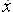Go to content
If you know what \displaystyle \frac{dy}{dx} is then you can figure out what y was by reversing the rules of differentiation. This is called integration. Functions can be integrated by application of a set of rules. Later in advanced mathematics these rules become a major topic of study. You must use the notation accurately.

## Summary/Background

Integration is the reverse of differentiation. The integral of x^p is written as \displaystyle\int x^p \, dx , which is read as "the integral with respect to x" and is given by \displaystyle \frac{1}{p+1}x^{p+1} + c, p \ne -1.
The constant c is known as the "constant of integration", and should always be included when integrating. Omitting the constant of integration is a common error in examinations. You may lose marks in exams if you omit it. You may also find that later parts of a question cannot be completed if this constant was omitted earlier on.Gottfried Leibniz (1 July 1646 - 14 Nov 1716) was a German mathematician who developed the present day notation for the differential and integral calculus though he never thought of the derivative as a limit. His philosophy is also important and he invented an early calculating machine.

The integral symbol ∫ was first used by Leibniz on October 29, 1675, in an unpublished manuscript. Several weeks later, on Nov. 21, he first placed dx after the integral symbol. Later in 1675, he proposed the use of the symbol in a letter to Henry Oldenburg, secretary of the Royal Society: "Utile erit scribi ∫ pro omnia, ut ∫l = omn. l, id est summa ipsorum l" [It will be useful to write ∫ for omn. so that ∫l = omn. l, or the sum of all the l's.] The first appearance of the integral symbol in print was in a paper by Leibniz in the Acta Eruditorum. The integral symbol was actually a long letter S for "summa."You can get a better display of the maths by downloading special TeX fonts from jsMath. In the meantime, we will do the best we can with the fonts you have, but it may not be pretty and some equations may not be rendered correctly.

## Glossary

### calculus

the study of change; a major branch of mathematics that includes the study of limits, derivatives, rates of change, gradient, integrals, area, summation, and infinite series. Historically, it has been referred to as "the calculus of infinitesimals", or "infinitesimal calculus".
There are widespread applications in science, economics, and engineering.

### constant

a value in a formula or equation that cannot change.

### constant of integration

the constant part of the indefinite integral of a given function

### derivative

rate of change, dy/dx, f'(x),, Dx.

### differentiation

The process of finding a derivative.

### integral

the anti-derivative

### integration

the process of finding an integral, the reverse process to differentiation.

### limit

the value that a function f(x) approaches as the variable x approaches a value such as 0 or infinity

### union

The union of two sets A and B is the set containing all the elements of A and B.

Full Glossary List

## This question appears in the following syllabi:

SyllabusModuleSectionTopicExam Year
AP Calculus AB (USA)4IntegrationRules-
AP Calculus BC (USA)4IntegrationRules-
AQA A-Level (UK - Pre-2017)C1IntegrationRules-
AQA AS Maths 2017Pure MathsIntegrationIntegration Basics-
AQA AS/A2 Maths 2017Pure MathsIntegrationIntegration Basics-
CBSE XII (India)CalculusIntegralsReverse process of differentiation-
CCEA A-Level (NI)C2IntegrationRules-
CIE A-Level (UK)P1IntegrationRules-
Edexcel A-Level (UK - Pre-2017)C1IntegrationRules-
Edexcel AS Maths 2017Pure MathsIntegrationIntegration Basics-
Edexcel AS/A2 Maths 2017Pure MathsIntegrationIntegration Basics-
I.B. Higher Level6IntegrationRules-
I.B. Standard Level6IntegrationRules-
Methods (UK)M9IntegrationRules-
OCR A-Level (UK - Pre-2017)C2IntegrationRules-
OCR AS Maths 2017Pure MathsIntegrationIntegration Basics-
OCR MEI AS Maths 2017Pure MathsIntegrationIntegration Basics-
OCR-MEI A-Level (UK - Pre-2017)C2IntegrationRules-
Pre-U A-Level (UK)5IntegrationRules-